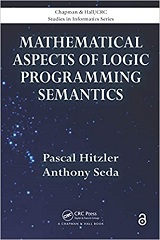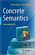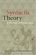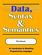Processing ......FreeComputerBooks.com Links to Free Computer, Mathematics, Technical Books all over the World

Mathematical Aspects of Logic Programming Semantics
Top Free Computer Networking Books 🌠 - 100% Free or Open Source!
• Title: Mathematical Aspects of Logic Programming Semantics
• Author(s) Pascal Hitzler, Anthony Seda
• Publisher: CRC Press; 1st edition (June 14, 2017); eBook (Open Acess by Taylor & Francis Group)
• Hardcover/Paperback: 304 pages
• eBook: PDF (305) and Read Online
• Language: English
• ISBN-10/ASIN: 1138114227/B00OD4KH4I
• ISBN-13: 978-1138114227Book Description

Covering the authors' own state-of-the-art research results, this book presents a rigorous, modern account of the mathematical methods and tools required for the semantic analysis of logic programs. It significantly extends the tools and methods from traditional order theory to include nonconventional methods from mathematical analysis that depend on topology, domain theory, generalized distance functions, and associated fixed-point theory. The authors closely examine the interrelationships between various semantics as well as the integration of logic programming and connectionist systems/neural networks.

The book covers topics spanning the period from the early days of logic programming to current times. It discusses applications to computational logic and potential applications to the integration of models of computation, knowledge representation and reasoning, and the Semantic Web. The authors develop well-known and important semantics in logic programming from a unified point of view using both order theory and new, nontraditional methods. They closely examine the interrelationships between various semantics as well as the integration of logic programming and connectionist systems/neural networks

• N/A

•Logic, Programming and Prolog, 2nd Edition (Ulf Nilsson, et al)

This book introduces major new developments in a continually evolving field and includes such topics as concurrency and equational and constraint logic programming.

•Inductive Logic Programming: Techniques and Applications

This book is an introduction to inductive logic programming (ILP), which aims at a formal framework as well as practical algorithms for inductively learning relational descriptions in the form of logic programs.

•An Introduction to Logic Programming Through Prolog (J. Spivey)

This is one of the few texts that combines three essential theses in the study of logic programming: logic, programming, and implementation. The techniques are illustrated by practical examples to explain how logic programming can be implented efficiently.

•Concrete Semantics: With Isabelle/HOL (Tobias Nipkow, et al.)

The book teaches the reader the art of precise logical reasoning and the practical use of a proof assistant as a surgical tool for formal proofs about computer science artefacts. It represents a formal approach to computer science, not just Semantics.

•Semantics with Applications: A Formal Introduction

This book provides a needed introductory presentation of the fundamental ideas behind Semantics, stresses their relationship by formulating and proving the relevant theorems, and illustrates the applications of semantics in computer science.

•Semantics in Action - Applications and Scenarios

This book is a nice blend of number of great ideas, theories, mathematical models, and practical systems in the domain of Semantics. It highlights highlights the state-of-the-art application areas in the domain of Semantics.

•Semantics - Advances in Theories and Mathematical Models

This book is a nice blend of number of great ideas, theories, mathematical models, and practical systems in the domain of Semantics. It highlights the advances in theories and mathematical models in the domain of Semantics.

•Syntactic Theory: A Formal Introduction (Ivan A. Sag, et al)

This is a textbook that makes it truly fun to teach introductory syntax. It is thoroghly data-driven and teaches the student to pay attention to empirical details and to find linguistic patterns and explanations for them.

•Data, Syntax and Semantics (J. V. Tucker, et al.)

This book is an introduction to the mathematical theory of programming languages. It is in tended to provide a first course, one that is suitable for all university students of Computer Science to take early in their education.

•Foundations of Fuzzy Logic and Semantic Web Languages

This book provides a rigorous and succinct account of the mathematical methods and tools used for representing and reasoning with fuzzy information within Semantic Web languages. The book focuses on the three main streams of Semantic Web languages.

Book Categories
 :All CategoriesTop Free BooksRecent BooksMiscellaneous BooksComputer EngineeringComputer LanguagesComputer ScienceData Science/DatabasesJava and Java EE (J2EE)Linux and UnixMathematicsMicrosoft and .NETMobile ComputingNetworking and CommunicationsSoftware EngineeringSpecial TopicsWeb Programming
Other Categories